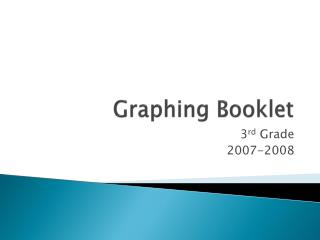DownloadDownload PresentationGraphing Booklet

Graphing Booklet

Download PresentationGraphing Booklet

- - - - - - - - - - - - - - - - - - - - - - - - - - - E N D - - - - - - - - - - - - - - - - - - - - - - - - - - -
Presentation Transcript

1. Graphing Booklet 3rd Grade 2007-2008

2. Bar Graphs • A graph that uses horizontal or vertical bars to represent various kinds of information.

3. Data Collection Sheet

4. Horizontal Bar Graph

5. Vertical Bar Graph

6. Line Graph • A graph formed by segments of straight lines that join the points representing certain data. Line graphs show changes over time.

7. Line Graph Example

8. Pictograph (Pictogram) • A graph drawn with pictures that represent the real objects.

9. Pictograph Example

10. Circle graph or Pie Chart • A circle graph is a pie chart. It is a graph that is created in a circle and shows the number, percent, or how something is divided.

11. Pie Chart